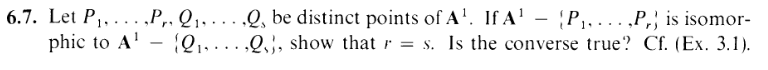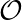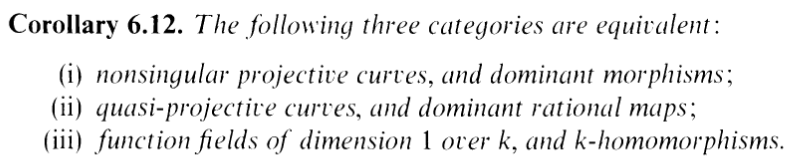I.6.7

9/10/2021Hello, reader! Welcome to SEEZON FEENALAY. Indeed, this section only turned out to have 7 exercises! Well, I skipped a few of them, but you'll have to give me a break. I've generally been doing more exercises per section than an actual math class would, rite? .... which is why I'm taking so long to get through these sections.... So there you go. gimme some leeway here, aight, yo?

This is one of those times I'm thankful I made this blog. I would have struggled a lot with this exercise, maybe not even been able to finish it, but I was able to largely thanks to my hard work back in 3.1b

I'm starting to self-insert as the girl in Fatalpulse doujinshi, it's great.

......................................................................

Anyway, I did create two very crafty lemmas during that exercise, which will help me out here. Actually, turns out I'm doing this exercise in a completely different way than our Queen intended, but whatevs. IF IT WORKS, IT WORKS. Here are the lemmas, btw:

LEMMA 1:

Given any two varieties X,Y where ϕ : X Y is an isomorphism, then the induced map ψ :(Y )(X) is an isomorphism.

LEMMA 2 (Regular funcs on open sets of A1 are rational):

If f(X) where X A1 is open in A1, then as a function on X, f = g∕h on X for g,h k[x] where h nowhere zero. Hence(X) = {rational functions on X}.

Okay, letting X = A1 -{P1,,Pr}, Y = A1 -{Q1,,Qs}. Let's assume wlg that r > s. Then thanks to my lovely LEMMA 1, if I show that(X)(Y ), I'm done. And I actually know what both of these rings look like thanks to LEMMA 2! Yaaaaaay. Here's the BIG TRICK: Based on LEMMA 2, we know that in(X), the elements x - Pi are invertible (whereas the elements x - Qi aren't: since we're told these points are distinct). And it's the opposite situation for(Y ). Now, if we had an isomorphism:

 ϕ :(X) →(Y )

First of all, we know that linear elements have to map to linear elements. "Ummm, how do you know that?" FUUUUUUUUUUUUUUUUUUUCK DUUUUUUUUUUUDE I DON'T FUCKIN KNOWWWWWW SO MANY EXERCISES LATELY HAVE RELIED ON THE ASSUMPTION THAT ISOMORPHISMS MAP LINEAR ELEMENTS TO LINEAR ELEMENTS LIKE REMEMBER I.5.14A AND OMGGGGGGG I SKIPPED 6.6C BUT LIKE THAT'S BASICALLY WHAT 6.6C IS SO LIKE IT'S LIKE SOOOOOOO COMMON AND I FUCKING SUCK AT PTOVING IT EACH TIME SO I'M JUST GOING TO ASSUME IT CUZ IT'S INTUITIVE AND AAAAAAAAHHHHH LEAVE ME ALONE LEAVE ME ALONE I JUST TOOK A 1.5 HOUR NAP AND IT'S 9PM AND I FEEL LIKE A ZOMBIE WHY DO I TAKE THESE STUPID IDIOTIC NAPS AND KILL MY ENERGY IDKKKKKKKKKKKKK HJELP ME SOMEONE..

Second of all, we know that invertible elements have to map to invertible elements. HENCE, x-R1 has to map to one of the x-Qi. BUT: of course we assumed r > s, so at some point we'll run out of x - Qis and injectivity will fail. But injectivity CAN'T fail cuz we need this to be an isomorphism. Contradiction, DUN.

Now, the rest of the exercise asks us if the converse is true. Lemme state the converse here:

If r = s, then A1 -{P1,,Pr}≃ A1 -{Q1,,Qs} (where the Pi,Qj are distinct)

Or a more concise way of stating it is

A1 -{P1,,Pr}≃ A1 -{Q1,,Qr} (where the Pi,Qj are distinct)

(again, I'll let the former be X and the latter be Y ) Now, let's do a little reading between the lines, shall we? The answer to this is "No". How do I know this? Because if the answer was "yes", then Queen Hartshorne wouldn't have asked it separately. Our Queen would have simply phrased the exercise as and if and only if statement. HENCE, the answer is "No". The converse is not true.

Now, can I make up an example to show the converse is not true? NOPE, LOL, BECAUSE I SUCK. FUUUUUUUUUUUUUUUUUUUUCK MYYYYYYYYYYYYYY ASSSSSSSSSSSSSSSSSSSSSS AAAAAAAAAAA THIS SECTION FUCKED ME IN THE ASS SO HARD. I ANSWERED "NO" AND ITS CORRECT I'M CONSIDERING THIS EXERCISE DONE LOLOLOLOL.

.

.

.

.

.

.

.

.

.

.

.

UPDATE: I... actually figured it out. This is crazy, but I actually did. I felt SO SUCKY when writing the paragraph above. Like, wow, I'm a failure and can't do anything. Even when I looked online, the solution just told me "the converse is not true when r > 3", with an explanation that didn't make any sense. Seriously, WTF? WHERE DID YOU GET 3 FROM?????? HOW AM I SUPPOSED TO KNOW SOMETHING BREAKS DOWN AT 3???? honestly i couldn't fathom why 3 would be such a special number here, and somewhere in the next few hours I basically gave up on this exercise. Obviously there's some advanced bs i'm missing or smething right?

Well, before going to bed I basically thought of it again, and then I thought of "3" again, AND THEN I FUCKING REALIZED SOMETHING. here's the thing: as I mentioned, I did the first half of this exercise in a different way you're supposed to. Remember this corollary from last time?The way you're supposed to do this exercise is by using (i) (ii). The isomorphism of X and Y (ii) yields an isomorphism of P1 with itself. i.e a birational equivalence of P1 with itself yields an automorphism of P1. And the key point is that this automorphism is an extension of the representative morphism of the rational map (as evident from the proof... which I didn't read till now lol). Okay, that was a lot of words, but THE POINT IS: if there were an isomorphism ϕ of X to Y , it extends to an automorphism of P1. So, how does this help with the converse?

well, last time was all about automorphisms of P1, rite? ACTUALLY, remember how I sorta humorously brought up Mobius transformations:

SECONDLY, for complex analysis fans, if k = , these are Mobius transformations! .... idk what else to say about that. HELP ME, starsofcurtains!

GUESS WHAT: THIS IS ACTUALLY THE KEY POINT (for my proof). ysee, I'm not quite a complex analysis fan. But one of the few things I learned in the past from my disastrous, embarassing attempt at ""self-study"" of the subject is this:

Given a set of three distinct points z1,z2,z3 on the Riemann sphere and a second set of distinct points w1,w2,w3, there exists precisely one Mobius transformation f(z) with f(zi) = wi for i = 1, 2, 3.

YOU GUYS SEE IT? SEE THOSE 3s? OK: Letting k = If we were extending an isomorphism

 ϕ : A1 -{P 1,…,Pr} → A1 -{Q 1,…,Qr}

to get an automorphism

 ϕ : P1 = ℂ ∪∞ → P1 = ℂ ∪∞

We know this has to be a Mobius transformation, thanks to 6.6. And, in particular, it's a Mobius transformation where the Pis map to the Qis. This is always possible if we only have 3 of each. BUT, as soon as we select 3 distinct points from each set, the resulting Mobius transformation (the extension) is FIXED (we're using uniqueness: the "precisely one" part here)–well, OKAY COMBINATORICS FELLAS, I know, there's technically errr i guess 3! different Mobius transformations, cause le order matters here, but there's ONLY 3! (!), a finite amount. So if we remove an extra point from each set, P4,Q4, and if this choice doesn't fit one of the preestablished Mobius transformations, there's no way to extend this isomorphism into an automorphism. SO WE ARE NOT GUARANTEED AN ISOMORPHISM FOR r > 3. THERE IT IS. YAAAAAAAAAAAAAAAAAAY.

Whew. Not bad for a seezon finale eh? I've had worse. I'll see you folks in the next section! Also i didn't even get myself cake last time... so maybe this time?